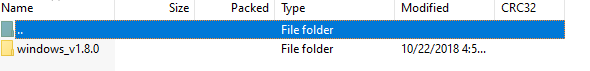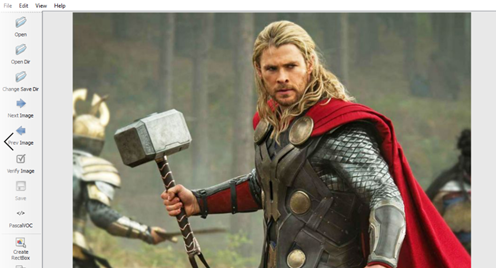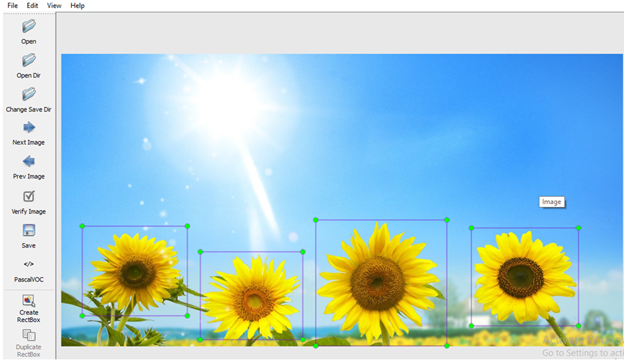top of page
Search

# Bounding Boxes for Character Recognition

Updated: Aug 7, 2021

A bounding box is an imaginary rectangle that are used to outline the object in a box as per as machine learning project requirement. They are the main outcomes of object detection model. The imaginary rectangle frame that surrounds an object in an image. Bounding boxes specifies position of object, its class as well as confidence that that tell us the chance of the object present at that location. Blue rectangle is bounding box that describe where our object (ironman) is located in imageBounding box contain two pairs of co-ordinate axis component i.e. one for upper left corner co-ordinate and other for lower right corner co-ordinate. conventions followed in representing bounding box:

1. Creating box with respect to top left and bottom right point of coordinates2. Creating the box with respect to its center, width, heightParameters used in this bounding box are :

• Class: represents the object inside the box. Eg. jimin in this case

• (x1, y1): x and y coordinate of the top left corner of the rectangle.

• (x2, y2): the x and y coordinate of the bottom right corner of the rectangle.

3. (xc, yc): x and y coordinate of the center of the bounding box. xc = ( x1 + x2 ) / 2 and yc = ( y1 + y2 ) /2

4. Width: width of the bounding box. width = ( x2 — x1)

5. Height: the height of the bounding box. height = (y2 — y1)

6. Confidence: Indicates probability of object present in that box. For example a confidence of 0.7 would indicate that there is a 70% chance that object actually exists in that box.

Model should predict the bounding box closed as ground truth as possible hence we have both the grand truth label and the predictions in bounding box format. Bounding boxes are one of the most popular image annotation techniques in deep learning. this method reduce costs and increase efficiency of annotation.

functions to perform conversion:

1. Conversion of upper-left and lower-right coordinates to center, width, height.

```def corner_to_center(boxes):

x1, y1, x2, y2 = boxes[:, 0], boxes[:, 1], boxes[:, 2], boxes[:, 3]
cx = (x1 + x2) / 2
cy = (y1 + y2) / 2
w = x2 - x1
h = y2 - y1
boxes = np.stack((cx, cy, w, h), axis=-1)
return boxes```

2. Conversion of center, width and height to upper-left and lower-right coordinates.

```def center_to_corner(boxes):
cx, cy, w, h = boxes[:, 0], boxes[:, 1], boxes[:, 2], boxes[:, 3]
x1 = cx – (0.5 * w)
y1 = cy – (0.5 * h)
x2 = cx + (0.5 * w)
y2 = cy + (0.5 * h)
boxes = np.stack((x1, y1, x2, y2), axis=-1)
return boxes```

Code for bounding box

`%matplotlib inline`

"""Sets the backend of matplotlib to the 'inline' backend so that the output of plotting commands is displayed inline within frontends like the Jupyter notebook, directly below the code cell that produced it. The resulting plots will then also be stored in the notebook document. """.

```from google.colab import drive
drive.mount('/content/drive')

```

above code is to take images saved in your google drive Once the Drive is mounted, you'll get the message “Mounted at /content/gdrive” , and you'll be able to browse through the contents of your Drive from the file-explorer pane. Now your Google Drive as if it was a folder in your Colab environment.

```%matplotlib inline
!pip install mxnet #to install mxnet in google coloab jupyter notebook
!pip install d2l #to install d21 in google coloab jupyter notebook
from mxnet import image, np, npx
from d2l import mxnet as d2l

npx.set_np()
d2l.set_figsize()
d2l.plt.imshow(img);```Defining bounding box to our object of interest

Suppose if we want identify two person Jisoo and V named as obj_1 and obj_2 respectively then we will define the bounding boxes of obj_1 and obj_2 in the image based on the coordinate information.

Let the coordinates of obj_1 are:

x1, y1, x2, y2

And coordinates of obj_2 are:

x1, y1, x2, y2

put the values of above coordinates

obj1 _bbox, obj2 _bbox =[30.0, 0.0, 350.0, 330.0], [440.0, 0.0, 690.0, 280.0]

put the value of x1,y1,x2,y2 for the object you choose to draw bounding boxes in # that coordinates

We will define a function name bbox_to_rectangle. It represents the bounding box in the bounding box format of the matplotlib package.

```def bbox_to_rectangle(bbox, color):
"""Convert bounding box to matplotlib format."""
return d2l.plt.Rectangle(xy=(bbox, bbox), width=bbox - bbox,
height=bbox - bbox, fill=False,
edgecolor=color, linewidth=2)
fig = d2l.plt.imshow(img)

```

""" axes.add_patch : in axes module of matplotlib library is used to add a Patch to the axes’ patches; return the patch."""

From below given image we can see the rectangle box on object 1 as well as object_2 . here obj_1 is jisoo and obj_2 is VBounding Boxes in Object Detection

Object detection has two components: image classification and object localization. In other words, to detect an object in an image, the computer needs to know what object we are looking for and where it is located in image. Image classification involves assigning a class label to an image, and object localization involves drawing bounding box around object of interest in an image these two process combines together and helps in object detection.

An annotator draw bounding boxes around other objects and label them. This helps train an algorithm to understand what object look like. Image annotation is the human-powered task of annotating an image with labels. To build and train any object detection model we need to have image dataset that is label or annotated

To label image we need to follow following steps:

Take dataset which you wish to train and test and make folder of it:

For example we wish to detect face of some famous character e.g.: BTS, avenger etc.

Make folder name data

As we are working on face detection project so in google drive make a folder name FaceDetection

Inside FaceDetection make folder of image

Inside image folder make folders of test image, test xml, train image, train xml.Download and upload 10 or more images of BTS and avengers in jpg format in train image folder and 5 images or more number of images in test image folder , try to take more number of images in dataset as more the number of image used more accurate result we get.Now generate xml file for each image of test and train image folders:

To lable image use following link:Click on windowsv_1.8.0Click on lableImg.exe. Then press run button.

After that click on open DirI have created another folder named image containing all images of test and train folder and saved it on desktop. By clicking on open dir (open directory) select that folder of image.

As I have saved that folder on desktop so first I will click on desktop option and then image folder and click on select folder option. After completing this step you can see image which we are going to label and at right bottom corner there appears the path of all the images which were saved in image folder.In order to label image press w from keyboard.now right click and drag the cursor to make a box around the object to be detected and label the image with a name then click on ok.Now to save this click on save option appearing on left as you save this you will get xml file of this image in image folder.When you will open the xml file you will see the detail of your image along with 4 coordinates xmin, xmax, ymin, ymax:By clicking on next you will be able to label next image are repeat this process until you generate xml file for all images. By labeling image and generating we get the four coordinates of our bounding boxes

Note: if you wish to label multiple object in single image for example image of sunflower as shown belowHere I have taken image of 3 bricks and to label all 3 of them now draw the box by following above steps (by pressing w and drag and make box on each brick and then save this as soon as you finish drawing box on each object)As you will save this you will get xml file of it.

Different Annotations Format

The bounding box has the following (x, y) coordinates of its corners: top-left is (x_min, y_min), top-right is (x_max, y_min), bottom-left is (x_min, y_max), bottom-right is (x_max, y_max). As you see, coordinates of the bounding box's corners are calculated with respect to the top-left corner of the image.

There are multiple formats of bounding boxes annotations. Each format uses its specific representation of bouning boxes coordinates. Albumentations supports four formats:

pascal_voc

Pascal VOC Bounding box :(x-top left, y-top left, x-bottom right, y-bottom right)

Pascal VOC provides standardized image data sets for object detection

Difference between COCO and Pacal VOC data formats will quickly help understand the two data formats

• Pascal VOC is an XML file, unlike COCO which has a JSON file.

• In Pascal VOC we create a file for each of the image in the dataset. In COCO we have one file each, for entire dataset for training, testing and validation.

• The bounding Box in Pascal VOC and COCO data formats are different

Pascal_voc is a format used by the Pascal VOC dataset. Coordinates of a bounding box are encoded with four values in pixels: [x_min, y_min, x_max, y_max]. x_min and y_min are coordinates of the top-left corner of the bounding box. x_max and y_max are coordinates of bottom-right corner of the bounding box

Albumentations

Like pascal_voc albumentations also uses four values [x_min, y_min, x_max, y_max] to represent a bounding box. But unlike pascal_voc, albumentations uses normalized values. To normalize values, we divide coordinates in pixels for the x- and y-axis by the width and the height of the image.

Let the coordinates of the bounding box are x1= 359, y1= 20, x2= 582, y2= 224

Hignt =638 width=850, then:

359 / 850, 20 / 638, 582 / 850,224 / 638] which are [0.422352, 0.031347, 0.684705, 0.351097].

Albumentations uses this format internally to work with bounding boxes and augment them.

COCO

COCO Bounding box: (x-top left, y-top left, width, height)

Coco is a format used by the Common Objects in Context COCO dataset.

In coco, a bounding box is defined by four values in pixels [x_min, y_min, width, height]. They are coordinates of the top-left corner along with the width and height of the bounding box.

YOLO

In yolo, a bounding box is represented by four values [x_center, y_center, w, h]. x_center and y_center are the normalized coordinates of the center of the bounding box. To make coordinates normalized, we take pixel values of x and y, which marks the center of the bounding box on the x- and y-axis. Then we divide the value of x by the width of the image and value of y by the height of the image. w and h represent the width and the height of the bounding box. They are normalized as well.

Class labels for bounding boxes

Along with coordinates, each bounding box should have an associated class label that tells which object is present inside the bounding box.

There are two ways to pass a label for a bounding box.

Let's say you have an example image with three objects: bird, sun and ball. Bounding boxes coordinates for those objects are [23, 74, 295, 388], [377, 294, 252, 161], and [333, 421, 49, 49].

1. passing labels with bounding boxes coordinates

bounding boxes with class labels will become [20, 75, 290, 308, 'bird'], [370, 299, 250, 150, 'sun'], and [330, 410, 50, 50, 'ball'].

There can be different types of class labels for example integer, string, or any other Python data type. For example,

integer values as class labels will look the following:

[20, 75, 290, 308, 18], [370, 299, 250, 150, 17], and [330, 410, 50, 50, 37].

We can use multiple class values for each bounding box, for example [20, 75, 290, 308, 'bird', 'animal'], [370, 299, 250, 150, 'sun', 'star'], and [330, 410, 50, 50, 37, ' ball', 'item'].

2. creating separate list and Passing labels for bounding boxes in it

For example, if we have three bounding boxes like [20, 75, 290, 308, 18], [370, 299, 250, 150], and [330, 410, 50, 50] we can create a separate list with values like ['bird', 'sun', 'ball'], or [18, 17, 37] that contains class labels for those bounding boxes.

Next, you pass that list with class labels as a separate argument to the transform function. Albumentations needs to know the names of all those lists with class labels to join them with augmented bounding boxes correctly. Then, if a bounding box is dropped after augmentation because it is no longer visible, Albumentations will drop the class label for that box as well. Use label_fields parameter to set names for all arguments in transform that will contain label descriptions for bounding boxes .

Read images and bounding boxes from the disk.

```image = cv2.imread("/path/to/image.jpg") # use to load image from given path
image = cv2.cvtColor(image, cv2.COLOR_BGR2RGB) # conversion of  BGR to RGB.```

Bounding boxes can be stored on the disk in different serialization formats: JSON, XML, YAML, CSV, etc. So the code to read bounding boxes depends on the actual format of data on the disk. After reading the data from the disk, next step is to prepare bounding boxes for Albumentations. Albumentations expects that bounding boxes will be represented as a list of lists. Each list contains information about a single bounding box. A bounding box definition should have four elements representing the coordinates of that bounding box. (either pascal_voc, albumentations, coco, or yolo). Besides four coordinates. to store additional information about the bounding box, such as a class label of the object inside the box by using definition of a bounding box may contain one or more extra values in it. Pass an image and bounding boxes to the augmentation pipeline and receive augmented images and boxes. There are two ways of passing class labels along with bounding boxes coordinates:

1. Pass class labels along with coordinates.

We can add a class label for each bounding box as an additional element of the list along with four coordinates :

```bboxes =
[20, 75, 290, 308, 'bird'],
[370, 299, 250,150, 'sun'],
[330, 410, 50, 50, 'ball'],
]```

Now pass an image and bounding boxes for it to the transform function and receive the augmented image and bounding boxes.

```transformed = transform(image=image, bboxes=bboxes)
transformed_image = transformed['image']
transformed_bboxes = transformed['bboxes']```

2. Pass class labels in a separate argument to transform.

Let's say you have coordinates of three bounding boxes

```bboxes = [
[20, 75, 290, 308],
[370, 299, 250, 150],
[330, 410, 50, 50]

]```

You can create a separate list that contains class labels for those bounding boxes:

`class_labels = ['bird', 'sun', 'ball']`

Then you pass both bounding boxes and class labels to transform. Note that to pass class labels, you need to use the name of the argument that you declared in label_fields when creating an instance of Compose in step

2. In our case, we set the name of the argument to class_labels.

```transformed = transform(image=image, bboxes=bboxes, class_labels=class_labels)
transformed_image = transformed['image']
transformed_bboxes = transformed['bboxes']
transformed_class_labels = transformed['class_labels']```

Note that label_fields expects a list, so you can set multiple fields that contain labels for your bounding boxes. So if you declare Compose like

```transform = al.Compose([
al.RandomCrop(width=450, height=450),
al.HorizontalFlip(p=0.5),
al.RandomBrightnessContrast(p=0.2),
], bbox_params=al.BboxParams(format='coco', label_fields=['class_labels', 'class_categories'])))```

We can use those multiple arguments to pass info about class labels, like

```class_labels = ['bird', 'sun', 'ball']
class_categories = ['animal', 'star', 'item']

transformed = transform(image=image, bboxes=bboxes, class_labels=class_labels, class_categories=class_categories)
transformed_image = transformed['image']
transformed_bboxes = transformed['bboxes']
transformed_class_labels = transformed['class_labels']
transformed_class_categories = transformed['class_categories']

```